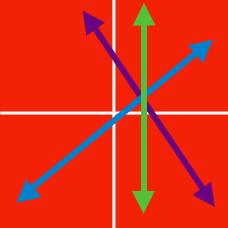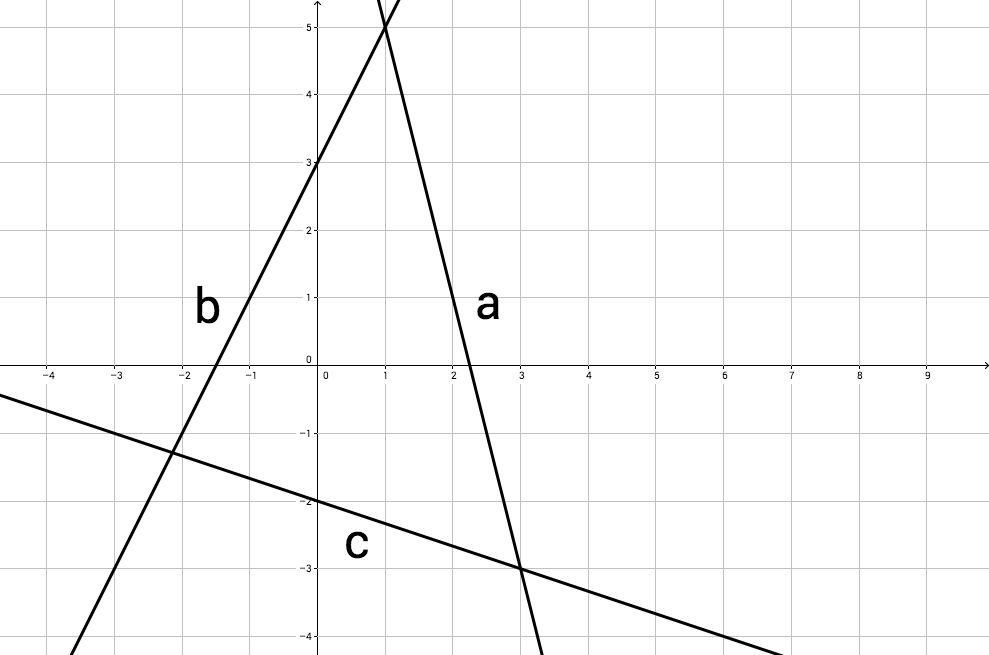Algebra

# System of Linear Equations Warmup

How many solutions are there for this system of equations? \begin{aligned} x + y = 4\\ x + y = 6 \end{aligned}Lines $a, b,$ and $c$ represent three different linear equations. What is the solution for the system of $a$ and $c?$

Two of these lines intersect at a point with positive coordinates. Which two?

A: $y = -3x -2$

B: $y = 3x -2$

C: $y = 0.5x +1$

D: $x = -3$

Find $xy$ if $2x + 4y = 2$ and $y - x = 5$.

How many $(x, y)$ pairs are solutions for both of these equations?

\begin{aligned} x + 2y &= 4 \\ 3x + 6y &= 12 \end{aligned}

×